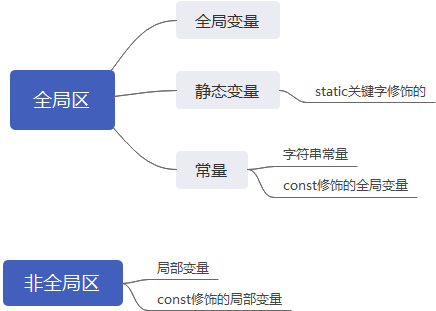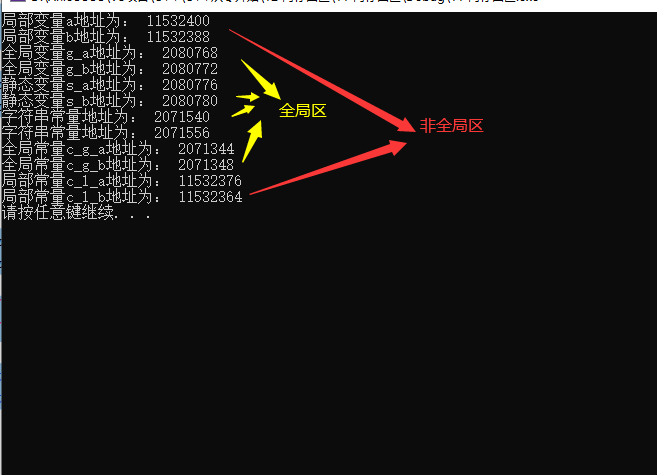# 内存四区(代码区、全局区、堆区、栈区）

C++在程序执行的时候，内存划分为4个区域

# 一、代码区

①代码区存放CPU执行的机器指令既程序汇编而成的二进制代码。
②代码区是共享的，对于频繁执行的程序，内存中只需要保存一份即可。
③代码区是只读的，只读的原因是防止程序意外修改了指令。

# 二、全局区``````#include <iostream>
using namespace std;

//全局变量
int g_a = 10;
int g_b = 10;

//全局常量
const int c_g_a = 10;
const int c_g_b = 10;

int main() {

//局部变量
int a = 10;
int b = 10;

//打印地址
cout << "局部变量a地址为： " << (int)&a << endl;
cout << "局部变量b地址为： " << (int)&b << endl;

cout << "全局变量g_a地址为： " << (int)&g_a << endl;
cout << "全局变量g_b地址为： " << (int)&g_b << endl;

//静态变量
static int s_a = 10;
static int s_b = 10;

cout << "静态变量s_a地址为： " << (int)&s_a << endl;
cout << "静态变量s_b地址为： " << (int)&s_b << endl;

cout << "字符串常量地址为： " << (int)&"hello world" << endl;
cout << "字符串常量地址为： " << (int)&"hello world1" << endl;

cout << "全局常量c_g_a地址为： " << (int)&c_g_a << endl;
cout << "全局常量c_g_b地址为： " << (int)&c_g_b << endl;

const int c_l_a = 10;
const int c_l_b = 10;
cout << "局部常量c_l_a地址为： " << (int)&c_l_a << endl;
cout << "局部常量c_l_b地址为： " << (int)&c_l_b << endl;

system("pause");

return 0;
}``````

结果验证：
从图中可以看出，只有局部变量和局部常量所在地址和其他变量相距很远，不在全局区之中。# 三、栈区

由编译器自动分配释放，存放函数的参数值，局部变量等。
注意事项：不要返回局部变量的地址，栈区开辟的地址由编译器自动释放。

``````#include <iostream>
using namespace std;

char* get_str(void)
{
printf("在子函数中：str = %s\n", str);
return str;
}

int main(void)
{
char* p = NULL;

p = get_str();
printf("在主函数中：p = %s\n", p);
printf("\n");
system("pause");
return 0;
}
``````两次打印的结果为什么不一致？原因是在执行完p = get_str();函数以后，指针变量p保存了数组str的首地址，但是由于数组定义在堆区，地址上的常量值已经被释放了，因此打印出来乱码！！

# 四、堆区

由程序员分配释放，若程序员不释放，则程序结束时由操作系统回收。
主要利用new关键字开辟堆区。

``````#include <iostream>
using namespace std;

int* func()
{
int* a = new int(10);
return a;
}

int main(void) {

int* p;
p = func();

cout << *p << endl;

system("pause");

return 0;
}``````首先在栈区定义了指针变量a，保存了堆区数据10的地址，而由于10定义在堆区需要有程序员手动释放或操作系统释放，因此在main函数执行完p = func();后指针变量p始终保存10的地址，因此可以打印出来！

new关键字用法

``````/*new 返回的是一个指针 */
int* a = new int(10);

/*利用delete释放堆区数据*/
delete a;

/*利用new创建数组*/
int* arr = new int;

/*利用delete释放数组*/
delete[] arr;``````

THE END